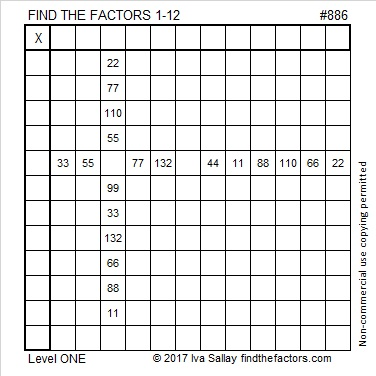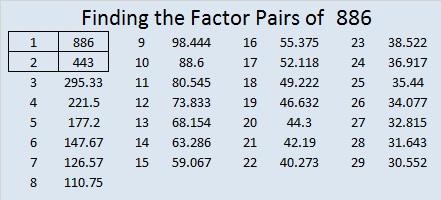# 886 and Level 1

886 is the sum of the sixteen prime numbers from 23 to 89.

886 is a palindrome in a couple of other bases:

• 12021 in BASE 5, because 1(5⁴) + 2(5³) + 0(5²) + 2(5) + 1(1) = 886
• 474 in BASE 14, because 4(14²) + 7(14) + 4(1) = 886Print the puzzles or type the solution on this excel file: 12 factors 886-896

• 886 is a composite number.
• Prime factorization: 886 = 2 × 443
• The exponents in the prime factorization are 1 and 1. Adding one to each and multiplying we get (1 + 1)(1 + 1) = 2 × 2 = 4. Therefore 886 has exactly 4 factors.
• Factors of 886: 1, 2, 443, 886
• Factor pairs: 886 = 1 × 886 or 2 × 443
• 886 has no square factors that allow its square root to be simplified. √886 ≈ 29.76575This site uses Akismet to reduce spam. Learn how your comment data is processed.